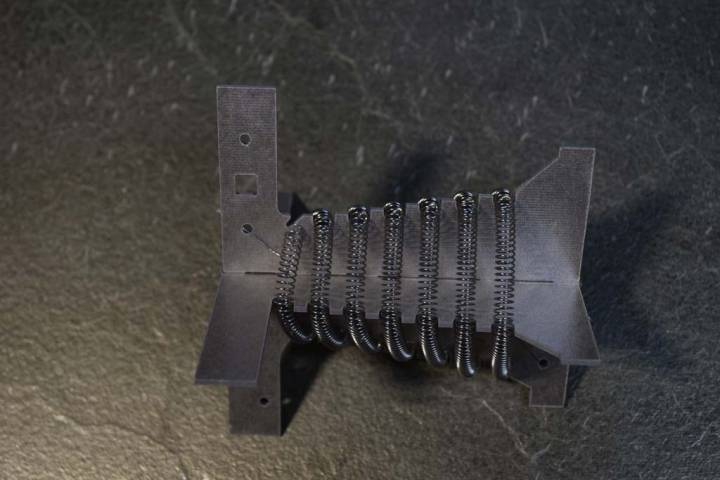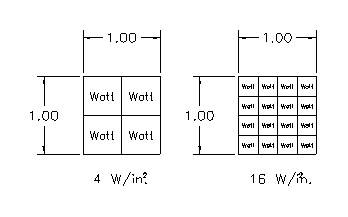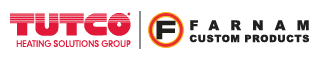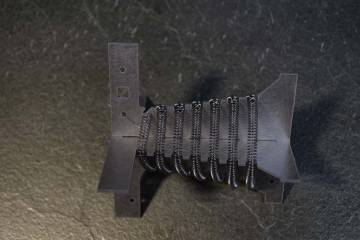Engineer Talk

# Why Does Watt Density Always Come Up When Discussing Heating Elements?## Overview

Watt density is a useful measure when considering the various types of heating elements available. Tutco-Farnam Custom Products manufactures open coil heating elements which are generally used to heat a gas flow, such as air or nitrogen. We are also manufacturers of surface heaters that heat by conduction. This discussion will pertain specifically to watt density and open coil elements.

## What is watt density?

Watt density is defined as the heating element power divided by the actively heated surface area of the element. If you were to pick up a heat transfer textbook, power/surface area is identified as the heat flux, but the term watt density has found common usage within the industry. In the United States, the units commonly used are [Watts]/[inches]². In other countries, [Watts]/[mm]² is often seen.

## Watt Density = Power / Surface Area = Heat Flux

Below is a mental picture I use when thinking about watt density. For a given area, a higher watt density will be more “dense” with wattage.## Why is watt density useful?

Watt density is a useful measure for predicting relative heating element temperature when comparing different alternatives. The operating temperature of the element is an important consideration for heat transfer efficiency and life.

There are three modes of heat transfer, conduction, convection, and radiation. Tutco-Farnam Custom Products’ open coil elements heat the air stream via forced convection. Forced convection takes place when a gas flow passes over a surface with a temperature different than the gas flow. The fundamental equation for convection heat transfer is Newton’s Law of Cooling.

## Q/A = h(ΔT) where;

Q = Power [Watt]

h = convection coefficient [Watt/( in.² · °F)]

h = f (V), function primarily of air velocity

A = surface area [in.²]

ΔT = temperature difference between surface and gas flow [°F]

ΔT = ( Tsurface - Tair)

The left side of this equation is watt density. On the right side of the equation, we have convection coefficient (h) multiplied by the temperature difference between surface and air flow (ΔT). The convection coefficient is primarily dependent on the velocity of the air flow. Unfortunately, the convection coefficient can be a bit complicated to calculate. The phenomenon’s complexity can be readily inferred from the numerous empirical equations used to calculate this quantity. In addition, in actual practice, the air velocities across a heating element are seldom uniform which further complicates calculations as well.

## Example of use

However, with this equation, we can still derive some good information with a minimum of effort. For example, I am a design engineer tasked with revising a product that uses a heater/blower combination. The existing product has a 100 CFM blower, and a 500W 120V CB heater with a watt density of 30 Watt/ in.². Marketing has determined that our company can gain more market share if a 1000W heater is used like their competitor’s. A 1000W 120V CB heater has a watt density of 45 Watt/ in.². I want to keep the same blower if at all possible. With the same blower, I can assume the air velocity is essentially the same, and therefore, (h) is the same for both cases. I also know that Tair the inlet air is room temperature whether I’m using a 500W or 1000W element. Knowing that I can instantly infer the 1000W element wire surface temperature, Tsurface, will operate at a higher temperature.

We can take this a step further with a minimum of math and information to estimate, “How much hotter?”. I don’t know what the coil surface temperature, Tsurface, of the existing 500W is. But if I take an educated guess, I can still estimate and get a feel for how much hotter the 1000W element will operate. I’m guessing the coil temperature, Tsur1, is somewhere between 600°F and 1000°F.

Q/A = h( Tsurface - Tair)

Case 1, 500W30 Watt/ in.² = h( Tsur1 - 70 °F)

If Tsur1 = 600°F

If Tsur1 = 1000°F

Case 2, 1000W

45 Watt/ in.² = h( Tsur1 - 70 °F)

Tsur2 = 865 °F, a 44% increase

Tsur2 = 1465 °F, a 47% increase

Since Tair is generally small relative to Tsurface, it becomes apparent an initial estimate in temperature increase can be had simply from the ratio of watt densities.

(Q/A)2 / (Q/A)1 ≈ Tsur2 / Tsur1

## Conclusion

The watt density of an open coil element quickly gives one a feel for how it is going to operate. Tutco-Farnam Custom Products generally limits the watt density for forced convection applications to 60 Watt/ in.². However, if the air velocity is high, or the air is directed directly on the coils, such as the Tutco-Farnam Custom Products Heat Torch family, watt densities as high as 110 Watt/ in.² are possible.

Check out some of our innovative open coil heater designs or tell us about your project.

Tutco-Farnam Custom ProductsCalculators
Calculators +